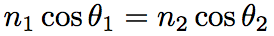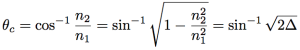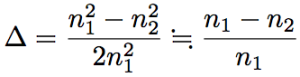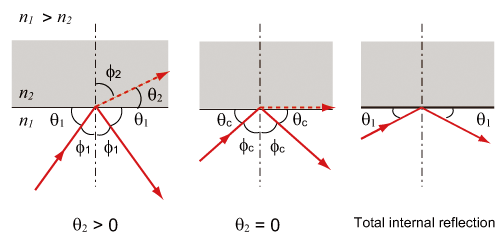In optical fiber, the total internal reflection of light occurs when the propagation angle, θ, is smaller than the critical angle, θc (total reflection angle). Now we consider propagation light  in optical fiber (see Fig.). In Fig., n1, and n2 is the refractive index of the core, and the clad, respectively, and the critical angle, θc, is the incident angle, φ1, at the refration angle of φ2=90 (θ2=0). Snell’s law is expressed as follows;therefore, the supplementary angle of the critical angle, θc, is defined by the following equation,where Δis relative refractive index difference, and is defined as follows.Generally, the relative refractive index difference, Δ, is represented by percentage (%). A normal optical fiber has Δ ~ 4 %. In the optical fiber, since the refractive index difference is small, the critical angle is large.Fig. Schematic picture of a critical angle and a total internal reflection.
The dashed line represents refraction light.
The solid line represents incident light and reflection light. φc is a critical angle.